# Link to Current (updated) notesNext: Integrating Factors, Exact Forms Up: Lecture_20_web Previous: Lecture_20_web
Subsections

# Ordinary Differential Equations from Physical Models

In engineering and physics, modeling physical phenomena is the means by which technological and natural phenomena are understood and predicted. A model is an abstraction of a physical system, often with simplifying assumptions, into a mathematical framework. Every model should be verifiable by an experiment that, to the greatest extent possible, satisfies the approximations that were used to obtain the model.

In the context of modeling, differential equations appear frequently. Learning how to model new and interesting systems is a learned skill--it is best to learn by following a few examples. Grain growth provides some interesting modeling examples that result in first-order ODES.

## Grain Growth

In materials science and engineering, a grain usually refers a single element in an ensemble that comprises a polycrystal. In a single phase polycrystal, a grain is a contiguous region of material with the same crystallographic orientation. It is separated from other grains by grain boundaries where the crystallographic orientation changes abruptly.

A grain boundary contributes extra free energy to the entire system that is proportional to the grain boundary area. Thus, if the boundary can move to reduce the free energy it will.

Consider simple, uniformly curved, isolated two- and three-dimensional grains.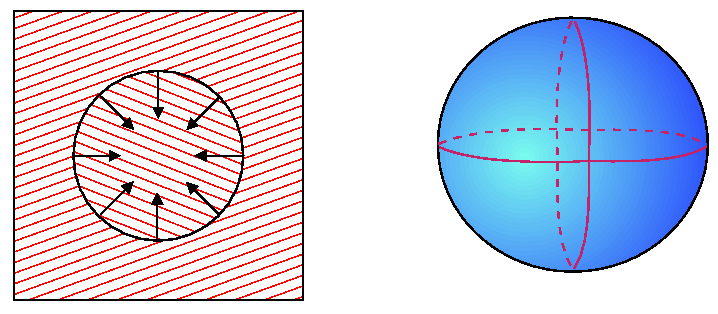A relevant question is how fast will a grain change its size assuming that grain boundary migration velocity is proportional to curvature?''

For the two-dimensional case, the rate of change of area can be formulated by considering the following illustration.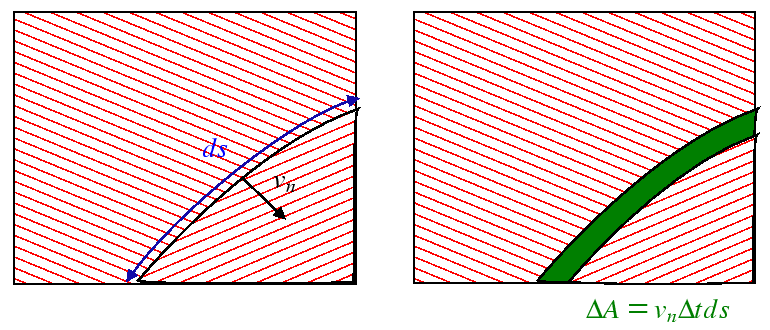Because for a circle, the curvature is the same at each location on the grain boundary, the curvature is uniform and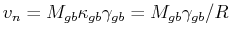. Thus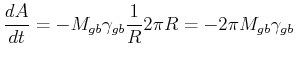(20-1)

Thus, the area of a circular grain changes at a constant rate, the rate of change of radius is: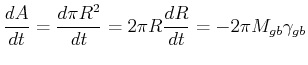(20-2)

which is a first-order, separable ODE with solution: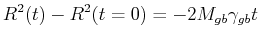(20-3)

For a spherical grain, the change in volume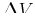due to the motion of a surface patch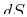in a time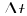is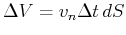. The curvature of a sphere is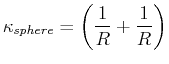(20-4)

Therefore the velocity of the interface is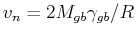. The rate of change of volume due to the contributions of each surface patch is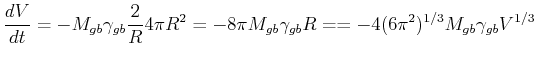(20-5)

which can be separated and integrated: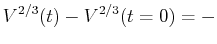constant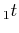(20-6)

or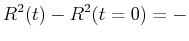constant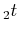(20-7)

which is the same functional form as derived for two-dimensions.

The problem (and result) is more interesting if the grain doesn't have uniform curvature.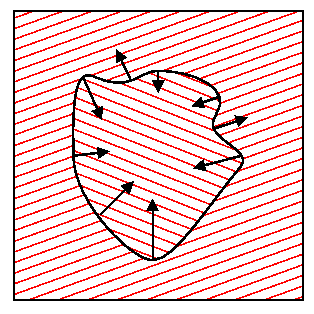However, it can still be shown that, even for an irregularly shaped two-dimensional grain,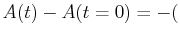const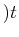.

© W. Craig Carter 2003-, Massachusetts Institute of Technology